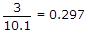# Electronics and Communication Engineering - Microprocessors

### Exercise :: Microprocessors - Section 3

16.

Assuming that k is an integer what would the result of following expression in C
k = 2* 3 / 5 + 4 / 4 + 8 - 2 - 4 / 8

 A. 8 B. 7 C. 7.5 D. zero

Explanation:

k = 1 + 1 + 8 - 2 - 0 = 8 because k is an integer.

17.

Which of the following is a valid real constant?

 A. 1281 B. 1281.0 C. KM D. 11821

Explanation:

It is the only one with decimal point.

18.

Which of the following is a valid real constant?

 A. -81.56 B. 73 C. 10254,1 D. 42

Explanation:

It is the only one with decimal and without comma.

19.

Consider the following program in C `int k float b ; k = 3 b = 10.1 k = k / b`

The final result would be k =

 A. 0.297 B. 0.2 C. 0 D. 3

Explanation:Since K is int hence answer is 0.

20.

The decimal equivalent of binary 1101.11 is

 A. 9.75 B. 13.75 C. 11.5 D. 11.75